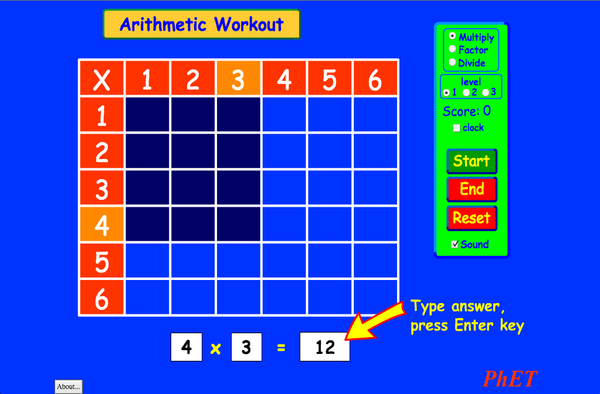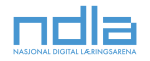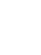# Arithmetic

Ev şêwekar hêj ji bo vî zimanê nehatiye wergerandin. Dîsa jî hun dikarin guhertoya bi Îngilîzî ya li jêr bixebitînin.DaxîneÇalkirin bigire Jibergirtiyekî çalak ya vê şêwekarê çal bikin Ji bo çalkirina jibergirtiyekî çalak ya vê şêwekarê vê qoda HTML bikar bînin. Hun dikarin firehî û bilindahiya şêwekarê bi guherandina nirxên "width" û "height" yên di HTML de biguherînin. Wêneyekî çalbikin ku bi tikandinê şêwekar bixebite
Bixebitîne
Ji bo nîşandana peyva "Bixebitîne" vê qoda HTML bikar bînin. Multiplication PhET tê piştgirîdan aliyêû mamosteyên mîna we.Back to HTML5 Version

### Naverok

• Multiplication

### Description

Remember your multiplication tables? Practice your multiplication, division, and factoring skills with this exciting game. No calculators allowed!

### Mînakên Armancên Fêrbûnê

• Explain how multiplication tables help understand multiplication, factoring, and division.
• Use an array model to understand multiplication, factoring, and division.
• Increase accuracy in multiplying, factoring and dividing.
• Develop multiple strategies for arithmetic problems.

### Standards Alignment

#### Common Core - Math

3.OA.A.1
Interpret products of whole numbers, e.g., interpret 5 × 7 as the total number of objects in 5 groups of 7 objects each. For example, describe a context in which a total number of objects can be expressed as 5 × 7.
3.OA.A.2
Interpret whole-number quotients of whole numbers, e.g., interpret 56 ÷ 8 as the number of objects in each share when 56 objects are partitioned equally into 8 shares, or as a number of shares when 56 objects are partitioned into equal shares of 8 objects each. For example, describe a context in which a number of shares or a number of groups can be expressed as 56 ÷ 8.
3.OA.A.4
Determine the unknown whole number in a multiplication or division equation relating three whole numbers. For example, determine the unknown number that makes the equation true in each of the equations 8 × ? = 48, 5 = _ ÷ 3, 6 × 6 = ?
3.OA.B.5
Apply properties of operations as strategies to multiply and divide.2 Examples: If 6 × 4 = 24 is known, then 4 × 6 = 24 is also known. (Commutative property of multiplication.) 3 × 5 × 2 can be found by 3 × 5 = 15, then 15 × 2 = 30, or by 5 × 2 = 10, then 3 × 10 = 30. (Associative property of multiplication.) Knowing that 8 × 5 = 40 and 8 × 2 = 16, one can find 8 × 7 as 8 × (5 + 2) = (8 × 5) + (8 × 2) = 40 + 16 = 56. (Distributive property.)
3.OA.B.6
Understand division as an unknown-factor problem. For example, find 32 ÷ 8 by finding the number that makes 32 when multiplied by 8.
3.OA.C.7
Fluently multiply and divide within 100, using strategies such as the relationship between multiplication and division (e.g., knowing that 8 × 5 = 40, one knows 40 ÷ 5 = 8) or properties of operations. By the end of Grade 3, know from memory all products of two one-digit numbers.
3.OA.D.9
Identify arithmetic patterns (including patterns in the addition table or multiplication table), and explain them using properties of operations. For example, observe that 4 times a number is always even, and explain why 4 times a number can be decomposed into two equal addends.
Version 2.03

### Teacher TipsDi rêbera mamosteyan (pdf) de rêwazên aliyê koma PhET ve hatine afirandin hene. ( PDF ).

### Teacher-Submitted Activities

Juego de Aritmética (Basado en Indagación)Trish Loeblein, traducido por Diana López HS
MS
Guided
ST
Bîrkarî
How do PhET simulations fit in my middle school program?Sarah Borenstein MS Yên din Kîmya
Zindîwerzanî
Fîzîk
Erdzanî
MS and HS TEK to Sim Alignment Elyse Zimmer MS
HS
Yên din Zindîwerzanî
Kîmya
Fîzîk
Math facts - Using the Multiplication Chart Tapan Sarkar MS Ez.geh Bîrkarî
Back to the Basics Nyetta Abernathy K-5
MS
HS
ST
Ez.geh
Bîrkarî
Discovering divisibility rules Stacy Larson HS
MS
Ez.geh Bîrkarî
곱셈 나눗셈 연습 SIM 사용설명서 이화국(Wha Kuk Lee) K-5
MS
PT
ST
PKêş
Fîzîk
Bîrkarî
ARITHMETIC_DIVISON Arlete Cabral K-5 Ez.geh Bîrkarî
OBJETO DE APRENDIZAGEM: ARITHMETIC Arlete Moura de Oliveira Cabral e Maria Cleide da Silva Barroso K-5 Guided Bîrkarî
Operações Aritméticas Fundamentais Georgyana Cidrão; Francisco Régis Vieira Alves K-5 Ez.geh
Guided
Bîrkarî
Ziman Downloador RunTips
Afrikayî All AfrikaansRekenkunde
Albanian All shqipAritmetika
Amarî All Amharicየሒሳብ ስሌቶች
Baskî All EuskaraAritmetika
Bosnî All BosanskiARITMETIKA
Chinese (China) All 中文 (中国)算术
Chinese (Taiwan) All 中文 (台灣)算數
Croatian All hrvatskiAritmetika
Danî All DanskAritmetisk
Dutch All NederlandsRekenen
Endonezkî All Bahasa IndonesiaAritmatika
Erebî All العربيةarithmetic
Erebî, Saudi Arabia All العربية (السعودية)arithmetic
Estonî All EestiAritmeetika
Farisî All فارسیحساب
Frensî All françaisArithmétique
Georgian All ქართულიარითმეტიკა
Germenî All DeutschRechnen
Grekî All ΕλληνικάΑριθμητικός
Hebrew All עבריתחשבון
Îspanî All españolAritmética
Îspanî (Meksîko) All español (México)Aritmética
Îspanî (Peru) All español (Perú)Aritmética
Italî All italianotabelline
Japanese All 日本語計算
Kazakh All KazakhАрифметика
Korean All 한국어곱셈 나눗셈 연습
Latvian All LatviešuAritmētika
Macarkî All magyarSzorzótábla játék
Macedonian All македонскиАРИТМЕТИКА
Marathi All मराठीअंकगणित
Polish All polskiArytmetyka
Portuguese (Brazil) All português (Brasil)Aritmética
Romanian All românăAritmetica
Russian All русскийАрифметика
Serbian All СрпскиАРИТМЕТИКА
Slovak All SlovenčinaAritmetika
Swahili All SwahiliHesabu
Swedish All svenskaMatteräkning
Tirkî All TürkçeAritmetik
Turkmen All TurkmenArifmetika
Vietnamese All Tiếng ViệtSố học
Windows Macintosh Linux
Microsoft Windows
XP/Vista/7/8.1/10
Macromedia Flash 9 or later
OS X 10.9.5 or later
Macromedia Flash 9 or later
Macromedia Flash 9 or later
Koma Pêşnûmakeriyê Danegehên Kesê-sêyemîn Spas Ji Bo